# Accretive mapping

Jump to: navigation, search

The great importance of the notion of "accretive mapping" consists in the fact that it allows one to treat many partial differential equations and functional differential equations from mathematical physics (such as the heat and wave equations) as suitable ordinary differential equations associated with accretive generators of suitable semi-groups in appropriate functional (Sobolev) spaces. This method, known as the semi-group approach, has significantly clarified and unified the study of many classes of partial and functional differential equations and has solved problems that had been left open by the previous classical methods.

Letbe a general Banach space with norm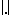. Ifis a bounded linear operator frominto itself, then the exponential formula below holds: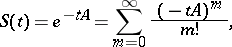as the series is convergent. Moreover, the function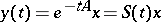is the unique strong solution to the Cauchy problem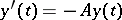,. Ifis unbounded, then the series above is not convergent, so the exponential formula makes no sense. However, ifis-accretive (see below and-accretive operator), then the so-called Crandall–Liggett exponential formula (1971) can be defined. Namely: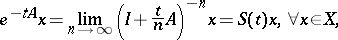as the limit above exists. Forlinear and unbounded, it is due to E. Hille and K. Yosida (who started these investigations in 1948). The one-parameter family of operators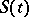defined byis said to be the semi-group generated by the (possible non-linear and multi-valued)-accretive mapping. The main difference in this unbounded case is that for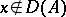, the function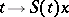is not differentiable. This is why the functionis said to be a mild (or generalized) solution to the Cauchy problem above.

Roughly speaking, accretive mappings acting inare generalizations of non-decreasing real-valued functions. More precisely, a mapping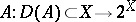is said to be accretive if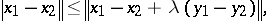(a1)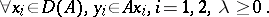Here,and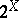stand for the domain ofand the family of all subsets of, respectively. Ifis a real Hilbert space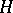with inner product, then (a1) is equivalent to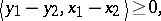(a2)It is now clear that for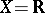(the set of all real numbers) anda single-valued function, accretivity ofis equivalent to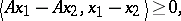(a3)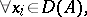i.e. to the classical definition "x1<x2 implies Ax1≤Ax2" forto be non-decreasing. The mappingis said to be dissipative if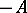is accretive.is said to be maximal accretive if it is accretive and if it has no accretive extensions.is said to be-accretive (or hyper-maximal accretive) if it is accretive and if the following range condition holds: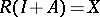, or, equivalently,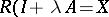, for all, wheredenotes the identity operator (cf. also-accretive operator).

In a normed space, "m-accretive" implies "maximal accretive" . The converse assertion need not be true. The first counterexample was constructed in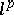by B.D. Calvert (1970). Moreover, A. Cernes (1974) has proven that even if bothand(the dual of) are uniformly convex (cf. Banach space), butis not a Hilbert space, then there are maximal accretive mappings which are not-accretive. However, it was proved by G. Minty (1962) that in Hilbert spaces, the notions of "m-accretive" and "maximal accretive" are equivalent. Note that in Hilbert spaces, "accretive" is also known as "monotone" .

The theory of accretive-type operators is also known as Minty–Browder theory. It has started with some pioneering work of M.M. Vainberg, E.M. Zarantonello and R.I. Kachurovski in the 1960s. As a significant example, consider the Laplace operator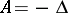in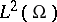with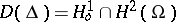, whereis a bounded domain of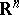with sufficiently smooth boundary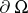. In view of the Green formula,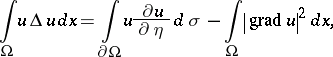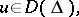it follows that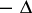is monotone. Moreover, for each, the elliptic equation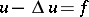has a unique solution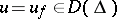, sois maximal monotone. H. Brézis has proved thatis actually the subdifferential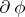of a lower semi-continuous convex functionalfromintowhich (according to a more general result of R.T. Rockafellar, 1966), is maximal monotone (accretive). It follows from the definition (a1) that ifis-accretive, then for every positive,is invertible and the operator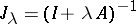is non-expansive (i.e., Lipschitz continuous of Lipschitz constant) on. The crucial importance of-accretive operators has already been pointed out above.

There is an extensive literature on this topic.

Finally, there is a second notion which also goes by the name "dissipative" (the Coddington–Levinson–Taro Yoshizawa dissipative differential systems). However, the notion of dissipative operators as defined above and that of dissipative systems are different.